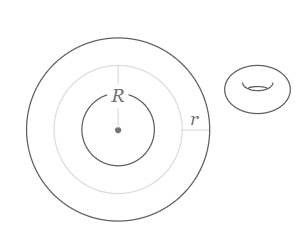## Torus Volume Surface Area Calculator

In geometry, a torus is a surface of revolution generated by revolving a circle in three-dimensional space about an axis coplanar with the circle.### Torus Volume Equation:

V = π2 * (R + r) * (R - r)2

### Torus Surface Area Equation:

S = π2 * (R2 - r2)